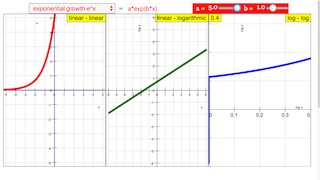Intro Page

y = f(x) in linear and logarithmiccoordinate systems

In a coordinate system with linear abscissa scaling and logarithmic ordinate scaling exponential functions appear as straight lines whose gradient is independent of a multiplicative factor.

Potency functions appear as straight lines in a twofold logarithmic coordinate system, with a slope, that indicates the grade of power. Twofold logarithmic coordinate systems emphasize the characteristics at small values of the abscissa by graphical spreading.

Log−linear graphs can only show positive values of the ordinate. Twofold logarithmic graphs are limited to positive values both of ordinate and abscissa. For some of the predefined functions an additive constant avoids cutoffs of negative ordinate values.

In the default setting three windows of different coordinate scaling show an exponential growth function with two free parameters a = 1 and b = 1. a and b can be varied by sliders. In the linear−linear scheme y = 1 is shown as a red line, and the two coordinate axes are shown as blue lines. The exact value of b appears in an editable number field, in which b is not limited to the range of the slider. Do not forget ENTER after a manual change!

The ComboBox allows selection of a predefined function, whose formula appears in the editable formula field. There you also can input any other function with up to two parameters a and b.

Power functions whose power grade is not an integer will result in imaginary values for negative x values. Only the branch for real x values can be shown. Integers are not easily defined with the slider. Instead, use the number field b to define integer power grades (as 2, 5, 25...)

Experiments

E 1:  In the default setting compare the three schemes for the exponential growth function. Derive the reason for the visual appearances from the formula.

E 2:  Change parameters a and b and observe the changes of the curves.. What happens at x = 0? Reflect the observation as result of the formula.

E 3:  Repeat the experiments for exponential damping.

E 4:  Choose the power function. Vary b and study the result in the twofold logarithmic scheme. Compare with the formula.

E 5:  If you choose b with the slider, you probably see just the positive branch in the linear−linear scheme of the potency. Why? Input positive and negative integers into the b text field and study which branches are now shown. Do not hesitate to also choose really high values for b.

E 6:  Study the appearance of the different periodic functions.

E 7:  Input your own formulas. Use proper factors to stay within the coordinate limits.

E 8: Try to formulate polynomials with several roots in the coordinate range. For some choose even, for others uneven maximum power.

Translations

Code Language Translator RunSoftware Requirements

SoftwareRequirements

 Android iOS Windows MacOS with best with Chrome Chrome Chrome Chrome support full-screen? Yes. Chrome/Opera No. Firefox/ Samsung Internet Not yet Yes Yes cannot work on some mobile browser that don't understand JavaScript such as..... cannot work on Internet Explorer 9 and below

CreditsDieter Roess - WEH- Foundation; Fremont Teng; Loo Kang Wee

[text]

Function in Linear and Logarithmic Coordinate Systems JavaScript Simulation Applet HTML5

Instructions

Function Box

Toggling the function box will give you their respective functions
and results in each panel.

(Exponential Growth e^x)

(Exponential Growth e^-x)

(Power Function)

(Sine)

(Sine Power)

(Power Sine)

(Hyperbolic Sine)

(Hyperbolic Cosine)

Editing the Functions

You can also edit the functions directing after picking a default state.

This can be done either by using the function field or the sliders in the
control panel.

Toggling Full Screen

Double clicking anywhere in the panel will toggle full screen.

Research

[text]

[text]

[text]

Testimonials (0)

There are no testimonials available for viewing. Login to deploy the article and be the first to submit your review!